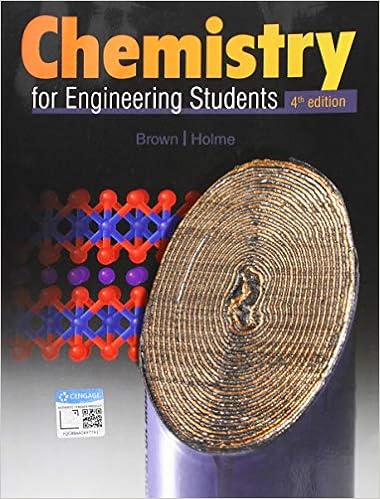# Determine the electron geometry eg and molecular

• Notes
• 16

This preview shows page 2 - 6 out of 16 pages.

##### We have textbook solutions for you!
The document you are viewing contains questions related to this textbook.The document you are viewing contains questions related to this textbook.
Chapter 7 / Exercise 7.56
Chemistry for Engineering Students
Brown/HolmeExpert Verified
9) Determine the electron geometry (eg) and molecular geometry (mg) of XeF2. A) eg=trigonal bipyramidal, mg=bent B) eg=linear, mg=linear C) eg=tetrahedral, mg=linear D) eg=trigonal bipyramidal, mg=linear E) eg=tetrahedral, mg=bent Answer:
D 10) Determine the electron geometry (eg) and molecular geometry (mg) of XeF4.
E 11) How many of the following molecules are polar? BrCl3CS2SiF4SO3
A
##### We have textbook solutions for you!
The document you are viewing contains questions related to this textbook.The document you are viewing contains questions related to this textbook.
Chapter 7 / Exercise 7.56
Chemistry for Engineering Students
Brown/HolmeExpert Verified
3 12) How many of the following molecules are polar? XeCl2COF2PCl4F SF6
B 13) How many of the following molecules are polar? XeO2SiCl2Br2C2Br2SeCl6A) 1 B) 4 C) 2 D) 3 E) 0 Answer:
C 14) How many of the following molecules are polar? PCl5COS XeO3SeBr2
D 15) Place the following in order of increasingdipole moment. I. BCl3II. BIF2 III. BClF2
III
<I
III
III
II
E
4 16) Place the following in order of decreasingdipole moment. I. cis-CHCl=CHCl II. trans-CHCl=CHCI III. cis-CHF=CHF
II
III
II
>I
II
A 17) Choose the compound below that contains at least one polar covalent bond, but is nonpolar. A) HCN B) CFC) SeBrD) IClE) Both B and C are nonpolar and contain a polar covalent bond. Answer:
4
4
3
B 18) Choose the compound below that contains at least one polar covalent bond, but is nonpolar.
2
2
5
2
C 19) Determine the electron geometry, molecular geometry and polarity of N2O (N central).
D 20) Determine the electron geometry, molecular geometry and polarity of XeF6.
E 21) Determine the electron geometry, molecular geometry and polarity of TeCl6. A) eg=octahedral, mg=octahedral, nonpolar B) eg=trigonal bipyramidal, mg=trigonal bipyramidal, nonpolar C) eg=octahedral, mg=square planar, polar D) eg=trigonal bipyramidal, mg=see-saw, polar E) eg=tetrahedral, mg=trigonal pyramidal, polar Answer:
A
5
•••